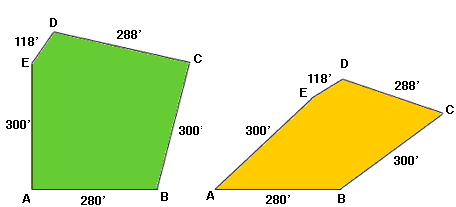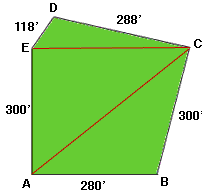Quandaries and Queries HELLO, I NEED HELP IN DETERMINING THE SIZE OF A PENTAGON, THIS IS A SECONDARY LEVEL QUESTION, I AM IN THE "OTHER " CATEGORY, MY NAME IS DANA one side is 280',from both ends of the 280 'section,we have 300' lengths,from one of these 300' sections we have a 118' length,which goes over to a length of 228',will this be enough info. or should i provide more. thanks Hi Dana, I have drawn below sketches of two possible lots that meet the requirements you sent.The lot on the left has a larger area than the lot on the right so the measurements you sent do not include enough information to determine the area. Somehow you need to tell me more about the "shape" of the lot. You could do this by telling me the angles at A, B, C, D, and E, but angles are difficult to measure. Another way to imagine the lot subdivided into triangles and tell me the lengths of the sides of the triangles. For example you could subdivide the lot as in the diagram below.If you tell me the lengths of AC and EC then I can find the areas of each of the three triangles and the area of the lot is the sum of the areas of the three triangles. Penny Go to Math Central